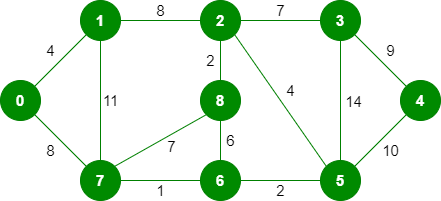# Kruskal’s Minimum Spanning Tree Algorithm | Factsprime

### Write a Modular C Programming code for ‎Kruskal’s Minimum Spanning Tree Algorithm DSA

Input Graph:The graph contains 9 vertices and 14 edges. So, the minimum spanning tree formed will be having (9 – 1) = 8 edges.

```After sorting:
Weight   Src    Dest
1         7      6
2         8      2
2         6      5
4         0      1
4         2      5
6         8      6
7         2      3
7         7      8
8         0      7
8         1      2
9         3      4
10        5      4
11        1      7
14        3      5```

CODE:

```#include<stdio.h>
#include<stdlib.h>
#include<string.h>

#define MAX 1000

int parent[MAX];

int find(int i)
{
while (parent[i] != i)
i = parent[i];
return i;
}

void union1(int i, int j)
{
int a = find(i);
int b = find(j);
parent[a] = b;
}

void kruskal(int graph[], int V, int E)
{
int i, j, u, v, min_cost = 0;

for (i = 0; i < V; i++)
parent[i] = i;

for (i = 0; i < E; i++)
{
u = find(graph[i]);
v = find(graph[i]);
if (u != v)
{
union1(u, v);
min_cost += graph[i];
printf("Edge %d - %d with weight = %d included in MST\n",
graph[i]+1, graph[i]+1, graph[i]);
}
}

printf("Minimum cost of MST = %d\n", min_cost);
}

int main()
{
int V, E, i, j;
int graph[MAX];

printf("Enter number of vertices and edges: ");
scanf("%d%d", &V, &E);

printf("Enter edges and their weights:\n");
for (i = 0; i < E; i++)
{
printf("Edge %d: ", i+1);
for (j = 0; j < 3; j++)
{
scanf("%d", &graph[i][j]);
}
}

kruskal(graph, V, E);

return 0;
}

```

OUTPUT

```Enter number of vertices and edges: 3
2
Enter edges and their weights:
Edge 1: 3
6
5
Edge 2: 2
1
6
Edge 3 - 2 with weight = 6 included in MST
Minimum cost of MST = 6

Process returned 0 (0x0)   execution time : 15.871 s
Press any key to continue.```

Please find some more codes of Loops, Condition Statements, 1D Arrays, 2D Arrays, Strings, Pointers, Data Structures, Files, Linked lists, Stacks, Queues, Binary Trees, MISC, Solved model question papers & Hacker Rank all solutions on the below page:

Top 100+ C Programming codes – KLE Technological University